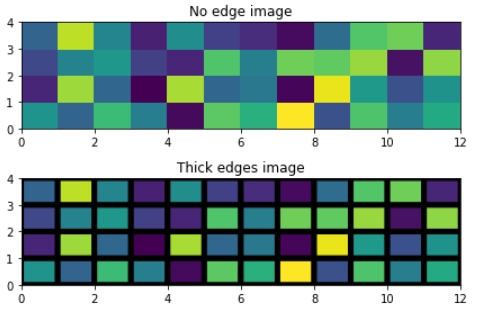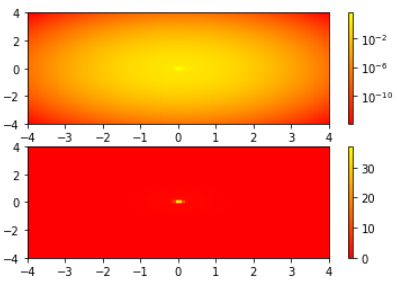# Matplotlib.pyplot.pcolor() function in Python

• Last Updated : 25 Nov, 2020

Matplotlib is the well-known Python package used in data visualization. Numpy is the numerical mathematics extension of Matplotlib. Matplotlib is capable of producing high-quality graphs, charts, and figures. Matplotlib produces object-oriented API for embedding plots into projects using GUI toolkits like Tkinter, wxPython, or Qt. John D. Hunter was the original developer of Matplotlib and it is distributed under a BSD-style license.

## matplotlib.pyplot.pcolor()

Matplotlib contains a wide range of functions that help in performing different tasks, one of them is matplotlib.pyplot.pcolor() function. The pcolor() function in the pyplot module of the Matplotlib library helps to create a pseudo-color plot with a non-regular rectangular grid.

Attention geek! Strengthen your foundations with the Python Programming Foundation Course and learn the basics.

To begin with, your interview preparations Enhance your Data Structures concepts with the Python DS Course. And to begin with your Machine Learning Journey, join the Machine Learning - Basic Level Course

Syntax: matplotlib.pyplot.pcolor(*args, alpha=None, norm=None, cmap=None, vmin=None, vmax=None, data=None, **kwargs)
Call Signature: pcolor([X, Y,] C, **kwargs)
Parameters:
C: Denotes a scaler 2-D array
X, Y: array_like, optional, coordinates of quadrilateral corners
cmap: str or Colormap, optional
norm: Normalize, optional
vmin, vmax: scaler, optional
edgecolors: {‘none’, None, ‘face’, color sequence}, optional
alpha: scaler, optional
snap: bool, optional
Other Parameters:
antialiaseds: bool, optional
**kwargs
Returns: The function returns a collection i.e matplotlib.collections.Collection

Note: In case of larger arrays, matplotlib.pyplot.pcolor() works very slow.

Below examples demonstrate the working of matplotlib.pyplot.pcolor() function:

Example 1: Generating images using pcolor() function

With the help of pcolor() function, we can generate 2-D image-style plots, as shown below

## Python3

 `# Demonstration of matplotlib function``import` `matplotlib.pyplot as plt``import` `numpy as np``from` `matplotlib.colors ``import` `LogNorm`` ` `Z ``=` `np.random.rand(``4``, ``12``)`` ` `fig, (ax0, ax1) ``=` `plt.subplots(``2``, ``1``)`` ` `c ``=` `ax0.pcolor(Z)``ax0.set_title(``'No edge image'``)`` ` `c ``=` `ax1.pcolor(Z, edgecolors``=``'k'``, linewidths``=``5``)``ax1.set_title(``'Thick edges image'``)`` ` `fig.tight_layout()``plt.show()`

Output:Example 2: Working of pcolor() with Log scale

## Python3

 `# Demonstration of matplotlib function``import` `matplotlib.pyplot as plt``import` `numpy as np``from` `matplotlib.colors ``import` `LogNorm`` ` `N ``=` `100``X, Y ``=` `np.mgrid[``-``4``:``4``:``complex``(``0``, N), ``-``4``:``4``:``complex``(``0``, N)]`` ` `# Image show that a low hump with a spike coming out.``# We need a z/colour axis on a log scale in order``# to watch both hump and spike.``Z1 ``=` `np.exp(``-``(X)``*``*``2` `-` `(Y)``*``*``2``)``Z2 ``=` `np.exp(``-``(X ``*` `10``)``*``*``2` `-` `(Y ``*` `10``)``*``*``2``)``Z ``=` `Z1 ``+` `50` `*` `Z2`` ` `fig, (ax0, ax1) ``=` `plt.subplots(``2``, ``1``)`` ` `c ``=` `ax0.pcolor(X, Y, Z,norm``=``LogNorm(vmin``=``Z.``min``(), vmax``=``Z.``max``()), cmap``=``plt.cm.autumn)`` ` `fig.colorbar(c, ax``=``ax0)`` ` `c ``=` `ax1.pcolor(X, Y, Z, cmap``=``plt.cm.autumn)``fig.colorbar(c, ax``=``ax1)`` ` `plt.show()`

Output:My Personal Notes arrow_drop_up